Consider a situation in which a set of 3D points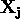is viewed by a set of cameras with matrices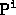. Denote by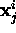the coordinates of the j-th point as seen by the i-th camera. We wish to solve the following reconstruction problem: given the set of image coordinatesfind the set of camera matrices,, and the pointssuch that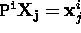. Without further restriction on theor, such a reconstruction is a projective reconstruction, because the pointsmay differ by an arbitrary 3D projective transformation from the true reconstruction.

If the image measurements are noisy then the equations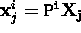will not be satisfied exactly. In this case we seek the Maximum Likelihood (ML) solution assuming that the measurement noise is Gaussian: we wish to estimate projection matrices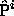and 3D points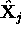which project exactly to image points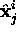as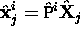, and also minimize the image distance between the reprojected point and detected (measured) image pointsfor every view in which the 3D point appears, i.e.\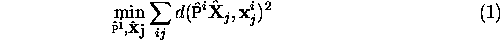where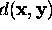is the geometric image distance between the homogeneous points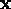and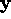. This estimation involving minimizing the reprojection error is known as bundle adjustment -- it involves adjusting the bundle of rays between each camera centre and the set of 3D points (and equivalently between each 3D point and the set of camera centres).

Bundle adjustment should generally be used as a final step of any reconstruction algorithm. This method has the advantages of being tolerant of missing data while providing a true ML estimate. At the same time it allows assignment of individual covariances to each measurement and may also be extended to include estimates of priors and constraints on camera parameters or point positions. In short, it would seem to be an ideal algorithm, except for the fact that: (i) it requires a good initialization to be provided, and (ii) it can become an extremely large minimization problem because of the number of parameters involved. We will discuss briefly these two points.

##### Iterative minimization.
Since each camera has 11 degrees of freedom and each 3-space point 3 degrees of freedom , a reconstruction involving n points over m views requires minimization over 3n + 11m parameters. In fact, since entities are often over-parametrized (e.g. using 12 parameters for the homogeneous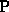matrix) this may be a lower bound. If the Levenberg--Marquardt algorithm is used to minimize then matrices of dimension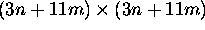must be factored (or sometimes inverted). As m and n increase this becomes extremely costly, and eventually impossible. There are several solutions to this problem:
1. Reduce n and/or m. Do not include all the views or all the points, and fill these in later by resectioning or triangulation respectively; or, partition the data into several sets, bundle adjust each set separately and then merge.

2. Interleave. Alternate minimizing reprojection error by varying the cameras with minimizing reprojection error by varying the points. Since each point is estimated independently given fixed cameras, and similarly each camera is estimated independently from fixed points, the largest matrix that must be inverted is the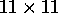matrix used to estimate one camera. Interleaving minimizes the same cost function as bundle adjustment, so the same solution should be obtained provided it converges to a global minimum, but it may take longer to converge. Interleaving is compared with bundle adjustment in W. Triggs, P. F. McLauchlan, R. I. Hartley, and A. Fitzgibbon, Bundle Adjustment for Structure from Motion. in Vision Algorithms: Theory and Practice, Springer-Verlag, 2000.

3. Sparse methods.

##### Initial solution.

If the problem is restricted to affine cameras then factorization gives a closed form optimal solution provided points are imaged in every view. Even with projective cameras there is an (iterative) factorization method available provided points are imaged in every view. If there is more information available on the data, for example that it is partly coplanar, then again a closed form solution is possible. Finally, hierarchical methods can be used for the case where points are not visible in every view.